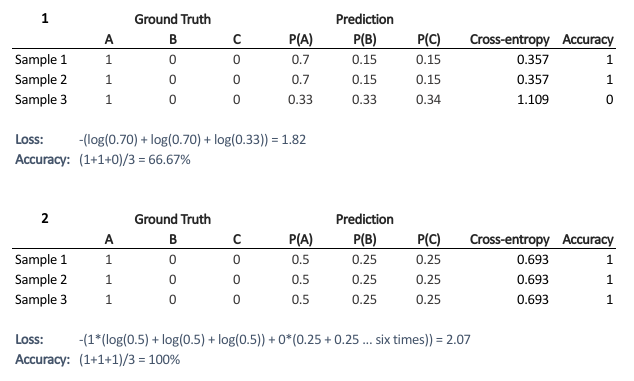# Loss vs Accuracy

Friday, December 7, 2018

A loss function is used to optimize the model (e.g. a neural network) you’ve built to solve a problem.

## Fundamentals

Loss is defined as the difference between the predicted value by your model and the true value. The most common loss function used in deep neural networks is cross-entropy. It’s defined as:

$\text{Cross-entropy} = -\sum_{i=1}^n \sum_{j=1}^m y_{i,j}\log(p_{i,j})$

where, $$y_{i,j}$$ denotes the true value i.e. 1 if sample i belongs to class j and 0 otherwise.
and $$p_{i,j}$$ denotes the probability predicted by your model of sample i belonging to class j.

Accuracy is one of the metrics to measure the performance of your model. Read about other metrics here. It’s defined as:

$Accuracy = \frac{\text{No of correct predictions}}{\text{Total no of predictions}}$

Most of the time you would observe that the accuracy increases with the decrease in loss. But, it may not be always true as in the given example.Now, the question is why does it happen?

It’s because accuracy and loss (cross-entropy) measure two different things. Cross-entropy loss awards lower loss to predictions which are closer to the class label. The accuracy, on the other hand, is a binary true/false for a particular sample. That is, Loss here is a continuous variable i.e. it’s best when predictions are close to 1 (for true labels) and close to 0 (for false ones). While accuracy is kind of discrete. It’s evident from the above figure.

## Conclusion

Given two models: one with high accuracy and high loss and other with low accuracy and low loss, which one would you choose? Here, the question you need to ask yourself before looking at accuracy or loss is What do you care about: Loss or Accuracy? If the answer is loss, then choose the model having lower loss, and if the answer is accuracy, choose the model with high accuracy.

References:

This page is open source. Improve its content!